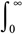A# gamma function

The gamma function is a generalization of the factorial function to the real line and to the complex plane. It is defined by:

Γ(n + 1) =xn e-x dx

If n is an integer, then Γ(n + 1) = n!